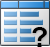# Create Grid Diagram using Open APIVisual Paradigm allows you to create the Grid Diagram for listing model elements in tabular form, structuring them into a table with elements as rows and properties as columns. The Grid Diagram can either be manipulated directly from the GUI, or through the use of the Open API. This article shows you how to develop a plugin yourself for creating a grid diagram. Use case models will be used for creating the grid diagram in this example as following:

## Create Model Elements for Showing in Grid

Firstly, let’s create some use case model elements for showing in grid. To create use case models:

```// Create couple of sample use cases and with different ranks
IUseCase uc1 = IModelElementFactory.instance().createUseCase();
uc1.setName("uc1");
uc1.setUcRank(IUseCase.UC_RANK_MEDIUM);```

Note that: Try to create few more use case models to make the experimental grid looks rich.

## Create Grid Diagram

Next we create a blank grid diagram. We use the DiagramManager.createDiagram method to create the grid diagram. Besides we also need to specify the model type where the grid is presenting using the IGridDiagramUIModel.setModelTypes method. In this example, the use case model will be used.

```// Obtain DiagramManager form ApplicationManager
DiagramManager diagramManager = ApplicationManager.instance().getDiagramManager();
// Create a new Grid Diagram
IGridDiagramUIModel gridDiagram = (IGridDiagramUIModel) diagramManager.createDiagram(IDiagramTypeConstants.DIAGRAM_TYPE_GRID_DIAGRAM);
// Specify the Grid Diagram for showing use case model
gridDiagram.setModelTypes(new String[] {IModelElementFactory.MODEL_TYPE_USE_CASE});```

## Create Columns

Now let’s create the columns for the grid. Each column will present a specific property of the model element. But before create the column we first have to create the IGridDiagramColumnContainer for storing the columns. Each project should have only one IGridDiagramColumnContainer and therefore we need to check whether it has already existed in the project. If it is exist, then we can simply reuse it, otherwise need to create a new one.

```// Locate/Create IGridDiagramColumnContainer for storing the columns
IGridDiagramColumnContainer colContainer = null;

// Obtain reference to our opening project
IProject project = ApplicationManager.instance().getProjectManager().getProject();

// Try to load the IGridDiagramColumnContainer from project
// if the resulting collection is not empty then we assume the IGridDiagramColumnContainer
// was found and we can reuse it. Otherwise we just create a new one
IModelElement[] containers = project.toAllLevelModelElementArray(IModelElementFactory.MODEL_TYPE_GRID_DIAGRAM_COLUMN_CONTAINER);
if (containers != null && containers.length > 0) {
colContainer = (IGridDiagramColumnContainer) containers;
} else {
colContainer = IModelElementFactory.instance().createGridDiagramColumnContainer();
}```

Once the IGridDiagramColumnContainer is ready, we can now start to create the columns using IGridDiagramColumnContainer.createGridDiagramColumn method.

```// Create column from IGridDiagramColumnContainer
IGridDiagramColumn colName = colContainer.createGridDiagramColumn();

// Specify the property for the column to present in grid
colName.setColumnValue(IGridDiagramColumn.PROP_NAME);```

Try to create few more columns for the properties you would like to see in the grid. After that set the columns for displaying in the grid.

```// Set the columns you would like to show into the grid
gridDiagram.setColumns(colContainer.toGridDiagramColumnArray());```

For this example, we just put all the columns we created into the grid. Sometimes, you might not want to display all columns in the grid. If this is the case, then you can also selectively store your favorite columns in an array for the inclusion in your grid.

## Show Up the Diagram

Finally, we can show up the grid diagram.

```// Show up Grid Diagram in UI
diagramManager.openDiagram(gridDiagram);```

## Sample Plugin

The sample plugin is attached to demonstrate how to create grid diagram for presenting the use cases. After you deploy the plugin into Visual Paradigm you can click the Generate Grid Diagram button to generate the grid.